## How To Get Answers and Steps For a Function of a Function Differentiation (Calculus) Problem Using The Calculator Encyclopedia

According to Wikipedia,

In mathematicsdifferential calculus is a sub-field of calculus concerned with the study of the rates at which quantities change. It is one of the two traditional divisions of calculus, the other being integral calculus, the study of the area beneath a curve.

Nickzom Calculator calculates function of a function for the following formats:

• (expression)n
• k(expression)n
• sin(expression)
• ksin(expression)
• cos(expression)
• kcos(expression)
• tan(expression)
• ktan(expression)
• sinh(expression)
• ksinh(expression)
• cosh(expression)
• kcosh(expression)
• cot(expression)
• kcot(expression)
• cosec(expression)
• kcosec(expression)
• In(expression)
• kIn(expression)
• loga(expression)
• kloga(expression)
• e(expression)
• ke(expression)
• a(expression)
• ka(expression)

k, a and in the formats above represents constants.

The expression represents a polynomial, quadratic or linear mathematical statement, such as:

• 3x + 5
• 4x2 + 2x + 1
• 2x3 + 7x + 12
• 5x4 + 3x3 + 4x2 + 12x + 15

So implementing the expressions and applying constant numbers here is a list of what the function of a function formats look like that can be solved by Nickzom Calculator and also display the steps (workings).

• (2x2 + 3x + 5)7
• 4(2x + 1)6
• sin(2x2 + 3x + 5)
• 2sin(3x + 5)
• cos(3x + 5)
• 2cos(3x + 5)
• tan(2x3 + 3x2 + 4x + 5)
• 3tan(7x + 6)
• sinh(2x4 + 12)
• 2sinh(3x + 10)
• cosh(x5 + 2x3 + 11)
• 3cosh(2x3 + 12x + 17)
• cot(2x + 1)
• 3cot(3x + 5)
• cosec(x + 12)
• 7cosec(2x + 7)
• In(x3 + 2x + 7)
• 2In(2x2 + 3x + 12)
• log3(3x + 7)
• 2log2(x2 + 4x + 8)
• e(3x + 5)
• 2e(2x + 3)
• 2(7x + 2)
• 7 . 2(6x + 7)

## Nickzom Calculator Calculates Permutation and Combination With Steps – The Calculator Encyclopedia

According to Wikipedia,

In mathematics, the notion of permutation relates to the act of arranging all the members of a set into some sequence or order, or if the set is already ordered, rearranging (reordering) its elements, a process called permuting.

In mathematics, a combination is a selection of items from a collection, such that (unlike permutations) the order of selection does not matter.

Getting answers and workings (steps) for permutation and combination problems in Nickzom Calculator – The Calculator Encyclopedia is very simple and I would very much love to show you how you can do this easily.

You can access Nickzom Calculator (The Calculator Encyclopedia) via any of these channels:

First and Foremost, proceed to the Calculator Map, under the Mathematics section click on Permutation|Combination.

Let’s begin with solving a problem on Permutation. I hope by now you do know the value of n and r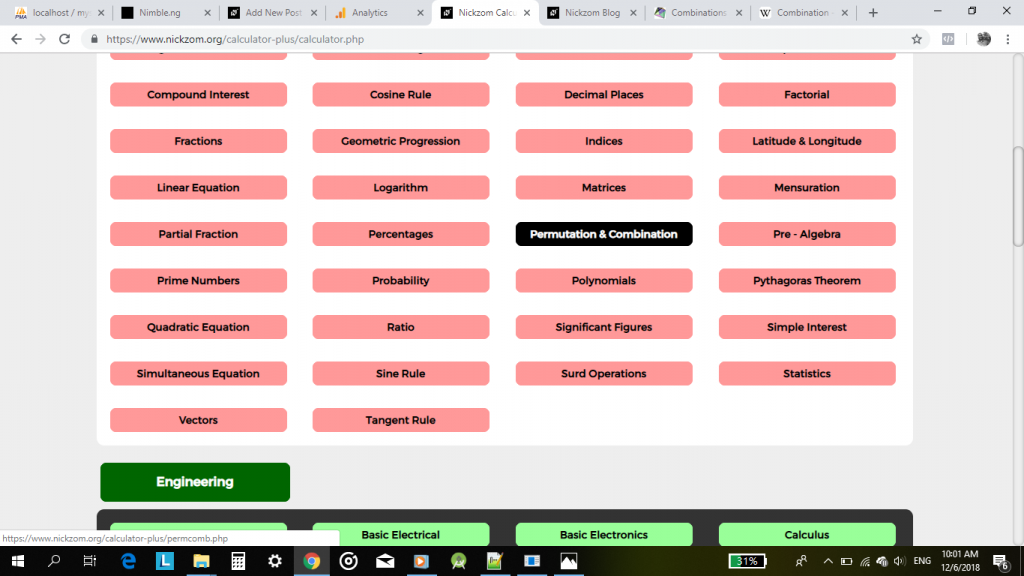## The Calculator Encyclopedia Performs Calculations on Binomial Series

According to Wikipedia,

In mathematics, the binomial series is the Maclaurin series for the function given by , where is an arbitrary complex number. Explicitly, and the binomial series is the power series on the right hand side of (1), expressed in terms of the (generalized) binomial coefficients.

Nickzom Calculator performs calculations on Binomial Series for both addition and subtraction.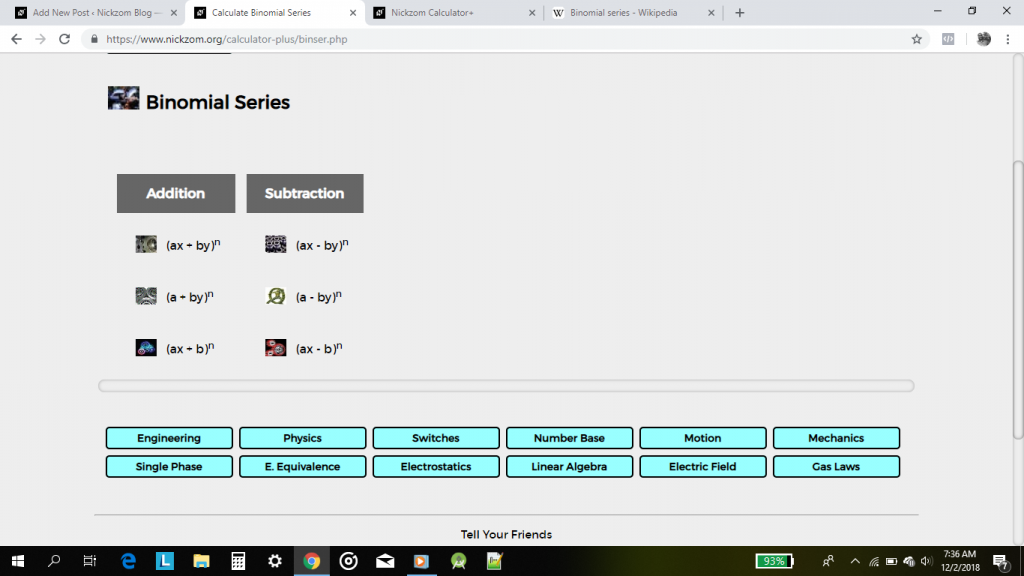## Nickzom Calculator Solves for Lowest Common Multiples and Highest Common Factor in Pre Algebra

According to Wikipedia,

In arithmetic and number theory, the least common multiplelowest common multiple, or smallest common multiple of two integers a and b, usually denoted by LCM(ab), is the smallest positive integer that is divisible by both a and b.] Since division of integers by zero is undefined, this definition has meaning only if a and b are both different from zero. However, some authors define LCM (a,0) as 0 for all a, which is the result of taking the LCM to be the least upper bound in the lattice of divisibility.

The LCM is the “lowest common denominator” (LCD) that can be used before fractions can be added, subtracted or compared. The LCM of more than two integers is also well-defined: it is the smallest positive integer that is divisible by each of them.

According to Math is Fun,

The highest common factor or greatest common divisor is the highest number that divides exactly into two or more numbers. It is the “greatest” thing for simplifying fractions!

Nickzom Calculator is capable of computing for a small or large range of numbers in determining the lowest common multiple or highest common factor

When it comes to lowest common multiple, popularly known as LCM for a range of numbers, it takes the multiples of all the numbers that can divide the range of numbers till the range of numbers are all 1.

When it comes to the highest common factor, popularly known as HCF or GCD, it takes the multiple of the numbers that can only divide through all the ranges of numbers simultaneously.

If you have been following this blog by now, you must have known that the first thing to do is?

Proceed to the Calculator Map

## Nickzom Calculator Calculates the Mean for Discrete and Continuous Data with Frequency under Statistics

Nickzom Calculator is capable of calculating a lot of problems on statistics and one of them is mean under measures of central tendency.

To this is very simple.

To access this functionalities in Nickzom Calculator, you need to purchase our professional version – Nickzom Calculator+ (The Calculator Encyclopedia). You can access this software via any of these channels:

Straight up go to Calculator Map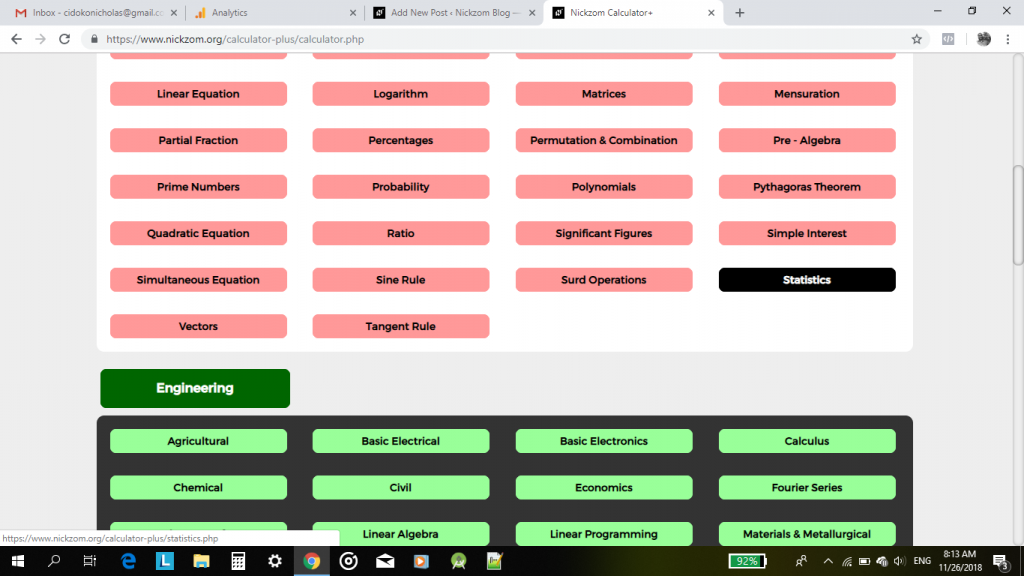Click on Statistics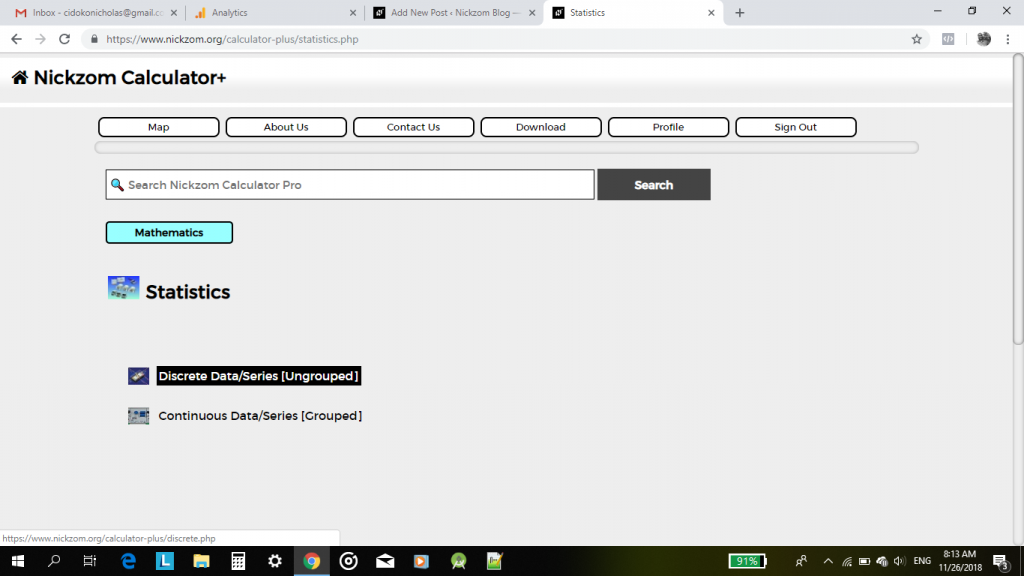Below, is a video displaying Nickzom Calculator solving the mathematical mean  of discrete and continuous data with and without frequencies.

## How to Get Answers of a 2 By 2 Matrix Linear Programming Maximization Problem Without Artificial Variables Using Nickzom Calculator

According to Google Dictionary,

Linear Programming is a mathematical technique for maximizing or minimizing a linear function of several variables, such as output or cost.

According to Wikipedia,

Linear programming (LP, also called linear optimization) is a method to achieve the best outcome (such as maximum profit or lowest cost) in a mathematical model whose requirements are represented by linear relationships. Linear programming is a special case of mathematical programming (also known as mathematical optimization).

You might be wondering how possible is it for there to be a calculator that can really solve linear programming calculations and show the workings at the same time. My dear, Nickzom Calculator does that for you at the very best of all capabilities.

Nickzom Calculator can not only solve a 2 by 2 matrix linear programming problem but can also solve for an m by n matrix problem. At the moment, Nickzom Calculator can handle:

• Maximization Without Artificial Variable(s)
• Minimization Without Artificial Variable(s)

Computations of Linear Programming with artificial variables is on the way and I do promise to inform you once it is integrated into the calculator encyclopedia via this blog.

Nickzom Calculator makes use of simplex method to compute the result for linear programming problems. There is an iterative computation of steps to obtain the final result which is when there is no further negative entry in the index/third/P row of which a basic solution emerges for x and y variables with all the other variables equal to 0. At this point, the optimal solution and optimal value is given.

## Mathematical Ratios Can Be Solved By Nickzom Calculator – The Calculator Encyclopedia

According to Math Is Fun,

A ratio says how much of one thing there is compared to another thing.

According to Wikipedia,

A ratio may be considered as an ordered pair of numbers, as a fraction with the first number in the numerator and the second as denominator, or as the value denoted by this fraction. Ratios of counts, given by (non-zero) natural numbers, denote positive rational numbers, and as such may occasionally result in natural numbers.

According to WikiHow,

Ratios are mathematical expressions that compare two or more numbers. They can compare absolute quantities and amounts or can be used to compare portions of a larger whole. Ratios can be calculated and written in several different ways, but the principles guiding the use of ratios are universal to all.

Nickzom Calculator+ (Professional Version) – The Calculator Encyclopedia solves ratio in mathematics for any number of fractional parts.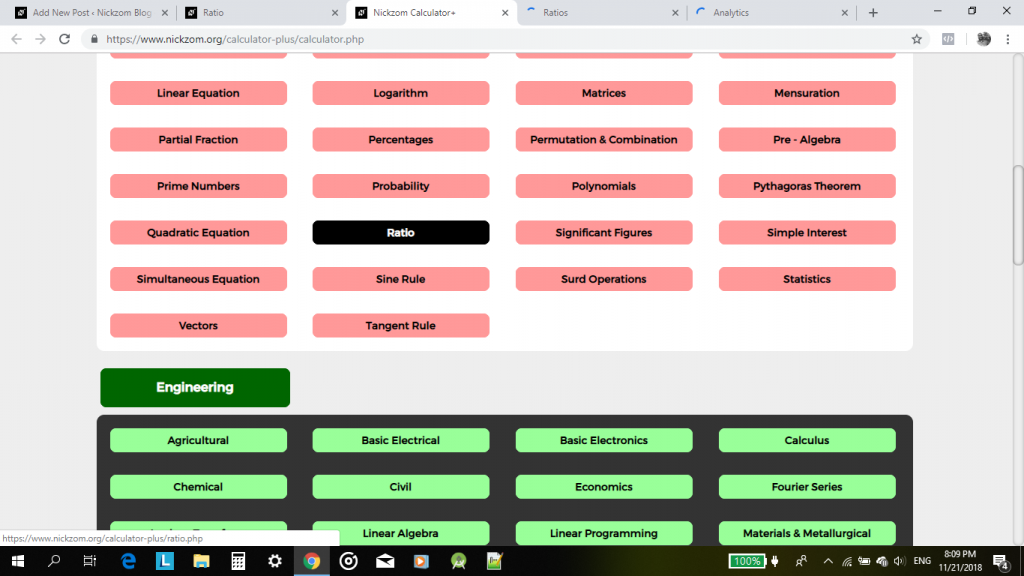For the calculator encyclopedia to be able to solve and present you a ratio. you must have to know the number of fractional parts you desire to compute the ratio for.

## The Calculator Encyclopedia Solves For Input and Output Reflection Coefficients, Power Gains and Mismatch Factors of S Parameters in Microwave Analysis

The knowledge of microwave analysis is a very added advantage for any Electronic Engineer out there. It makes one better understand the networking system of a two port in relation to S parameter.

According to Wikipedia, the S Parameters describe the electrical behavior of linear electrical networks when undergoing various steady state stimuli by electrical signals.

Luckily, Nickzom Calculator, the calculator encyclopedia is happy to take care of all calculations dealing with S Parameter (Two Port Network) for you and also show you a step by step guide (workings) on how to solve this problem.

For the purpose of this post, I would show you how Nickzom Calculator solves for the following:

• Input and Output Reflection Coefficients
• Various Power Gains
• Mismatch Factors

First of all, you have to have access to this awesome software package.

One can access Nickzom Calculator+ (Professional Version) – The Calculator Encyclopedia via any of these channels:

Once you have got access to the software package, please proceed to the Calculator Map then scroll to Engineering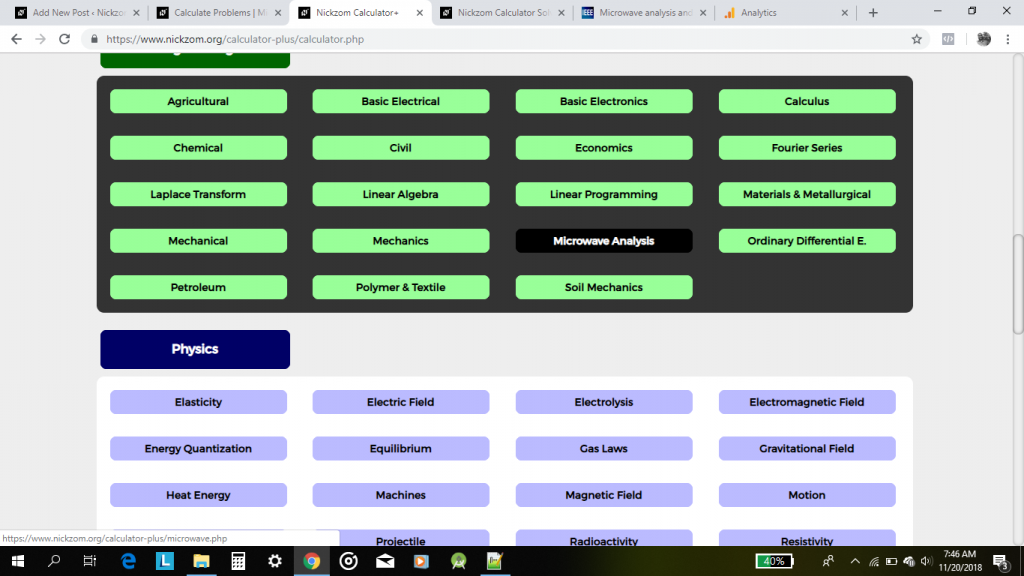## Nickzom Calculator Solves Linear Algebra

Linear Algebra is a matrix based mathematical computation analysis.

According to Wikipedia,

Linear algebra is central to almost all areas of mathematics. For instance, linear algebra is fundamental in modern presentations of geometry, including for defining basic objects such as linesplanes and rotations. Also, functional analysis may be basically viewed as the application of linear algebra to spaces of functions. Linear algebra is also used in most sciences and engineering areas, because it allows modeling many natural phenomena, and efficiently computing with such models. For nonlinear systems, which cannot be modeled with linear algebra, linear algebra is often used as a first-order approximation.

Nickzom Calculator solves 8 major sections of Linear Algebra such as:

• Matrix Arithmetic
• Determinant of a Square Matrix
• Minors and Cofactors of a Square Matrix
• Transpose of a Matrix
• Adjoint of a Square Matrix
• Inverse of a Square Matrix
• Eigenvalues and Eigenvectors of a Square Matrix
• Simultaneous Equation using Inverse Method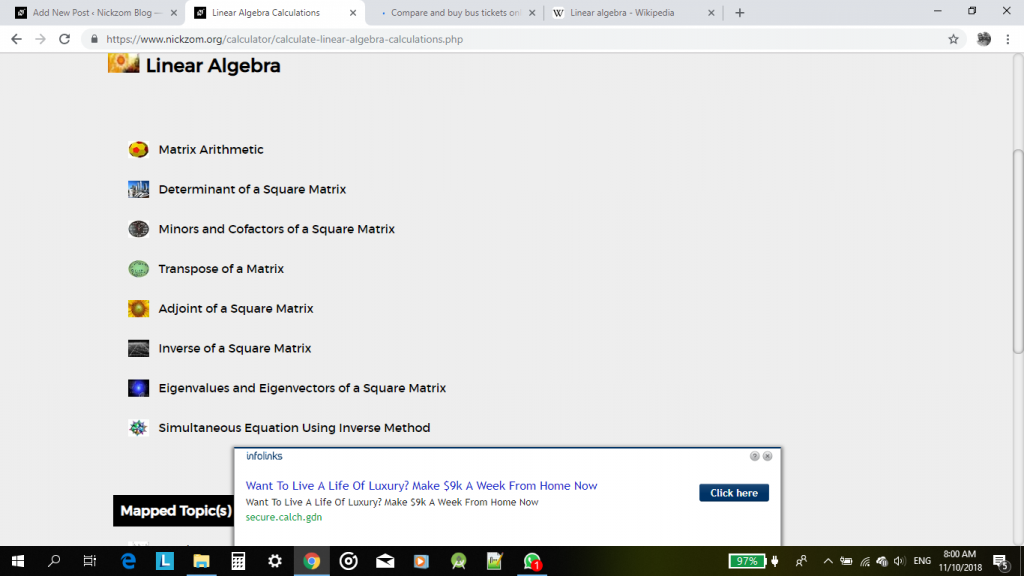Under, Matrix Arithmetic, there are 6 sub sections which are:

• Addition of Matrices
• Subtraction of Matrices
• Multiplication of a Matrix by a Scalar
• Multiplication of Two Matrices
• Division of a Matrix by a Square Matrix

## Mathematical Surd Operations can be Solved by Nickzom Calculator – The Calculator Encyclopedia

According to Laerd Mathematics,

Surds are numbers left in root form (√) to express its exact value. It has an infinite number of non-recurring decimals. Therefore, surds are irrational numbers.

According to Math is Fun,

• When it is a root and irrational, it is a surd
• Not all roots are surds

Nickzom Calculator solves calculations on surd and these calculations are categorized into:

• Surd Simplification A
• Surd Simplification B
• ‘Binary’ Surd Addition and Subtraction
• ‘Tenary’ Surd Addition and Subtraction
• Surd Multiplication
• Surd Division
• Surd Expansion
• Surd RationalizationNickzom makes use of a certain procedure to help the user easily input the surd values which is clearly represented in the image below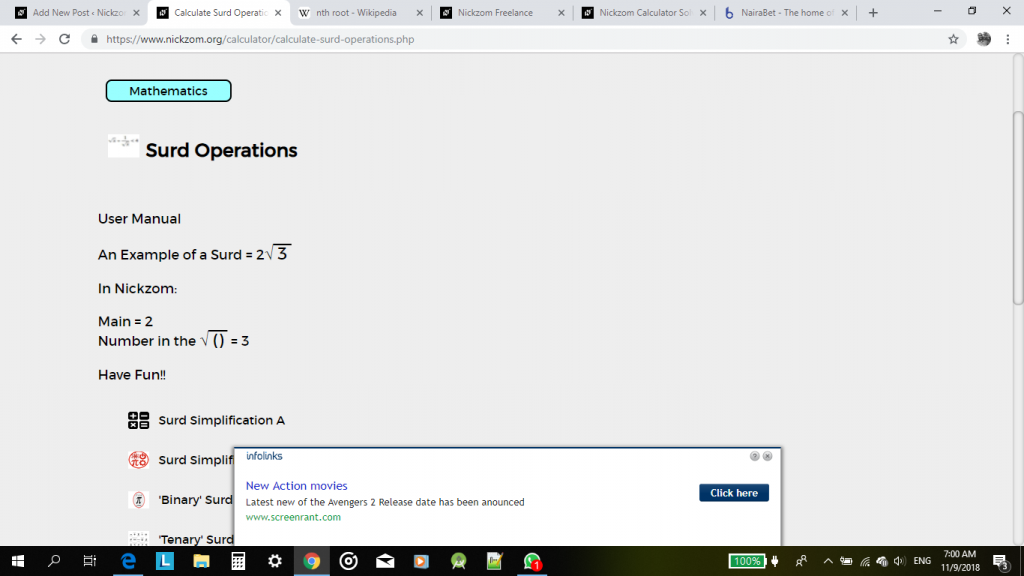For the purpose of this post, we would be handling a step by step guide on how to solve surd rationalization using Nickzom Calculator.

One can access Nickzom Calculator via any of these channels:

Now, click on Surd Rationalization, then enter the values appropriately using the user manual format.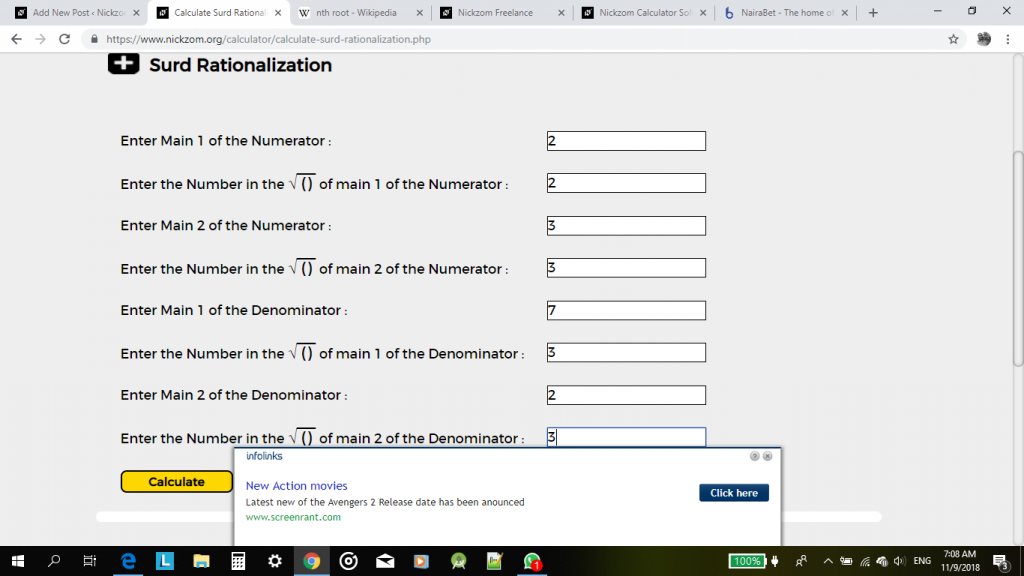Now, click on calculate to display the result.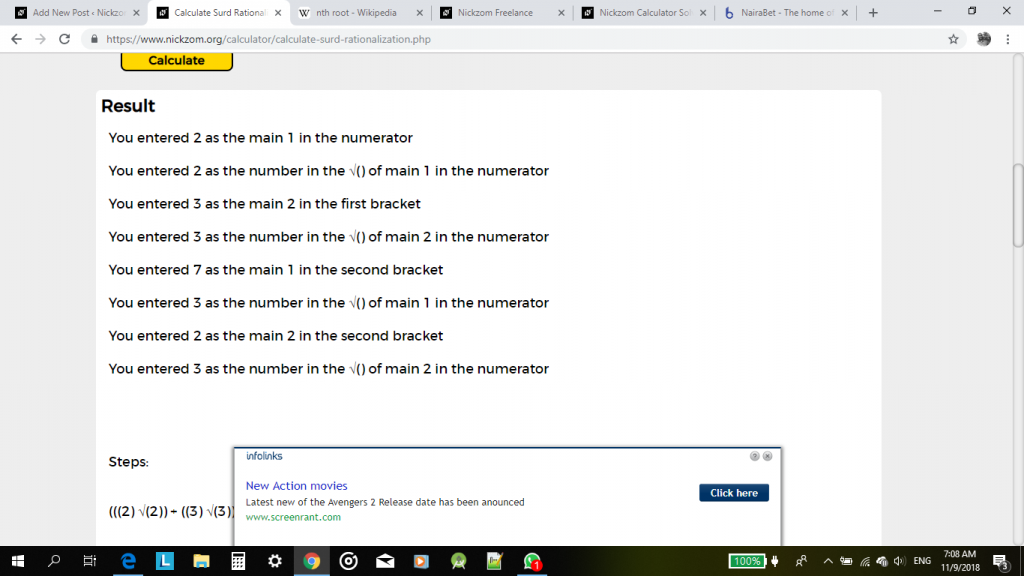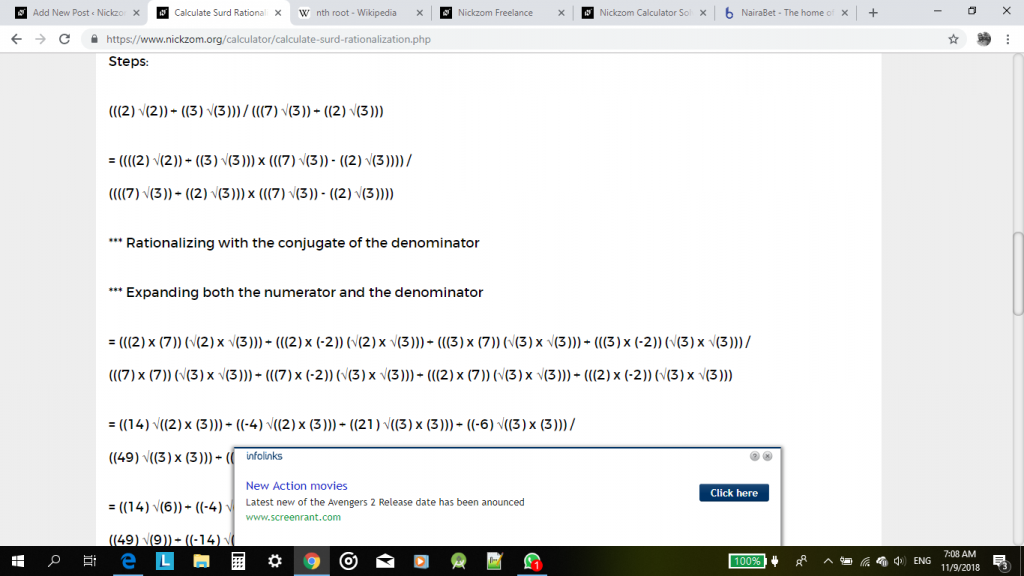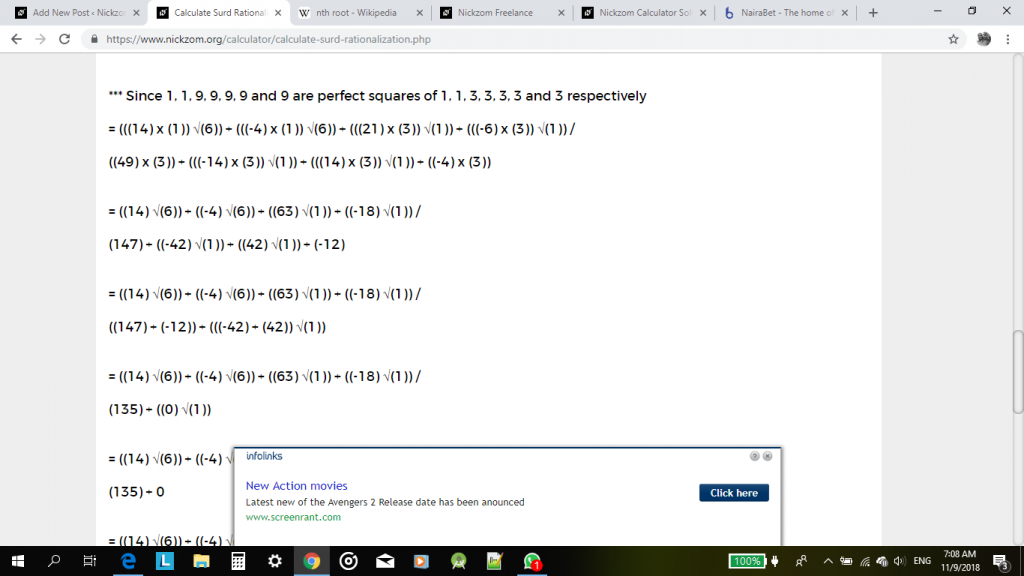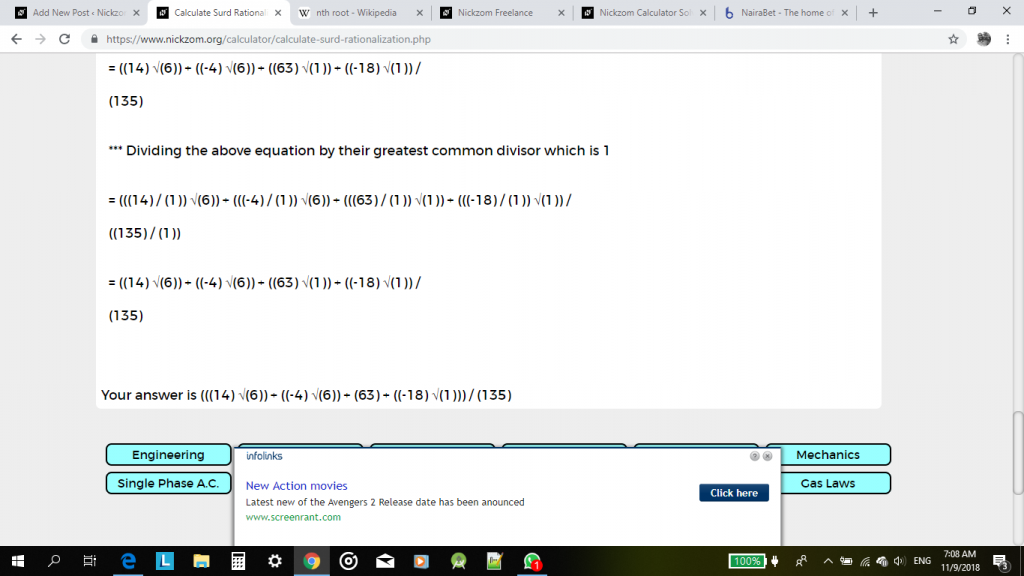I have seen that this steps and procedures might me cumbersome and a bit confusing for a reader or user. Therefore, an update would be coming soon with a new format of solving surd operations that can be easily understood and analysed. For now, with a bit of effort it could be understood or interpreted too.

Watch Out for the Update, Guys!